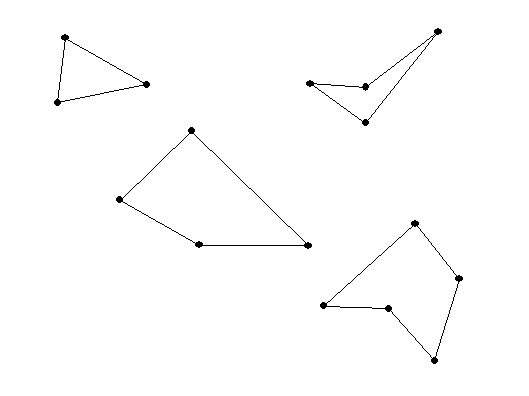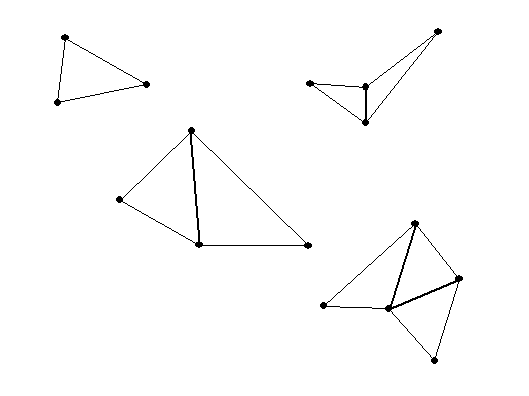Tuesday, September 9

Cutting and reassembling polygons.
Making Dissection Puzzles:

• More on measurements of angles and areas of polygons.

•     Discuss: 1.2 Ex. 4, 5, 6   and 7!

Consider a polygon with n sides.

When n = 3 this is a triangle, n=4, a quadrilateral, or when n= 5, a pentagon.The sum of the measures of the interior angles of a triangle is 180 degrees.
Question:What about a quadrilateral? and a pentagon?  or an n sided polygon  ( an "n -gon")?From the figure we can see that for a quadrilateral (n =4), which can be dissected into two triangles,
the sum is 2*180= 360 degrees.
And for a pentagon (n=5) which can be dissected into 3 triangles, the sum is 3*180=540 degrees.

In general: the sum of the interior angles in a n sided polygon is (n-2)* 180 degrees.

A regular  polygon is a polygon where the sides are all of equal length and the angles are all congruent (or of equal measure).

Question: what is the measure of an individual angle in a regular polygon with n sides?

For a triangle, the individual angle is 180/ 3 = 60 degrees.
For a square, the individual angle is 360 / 4 = 90 degrees.
For a regular pentagon.... 540/5=108  degrees.

Now for a HEXAGON (6 sides) the sum of the angles is (6-2)* 180= 4*180 = 720 degrees.
So ... for a REGULAR HEXAGON,  the individual angle is  720/6= 120 degrees.

In general: the individual angle for a regular polygon with n sides is (n-2)*180/n degrees.
This can be expressed in other ways using algebra:
(n-2)*180/n = [180 n - 360] / n = 180 - 360/n.
1. Dissection of the plane--- Tilings of the plane.
2. Activity: Tile plane with playing cards.